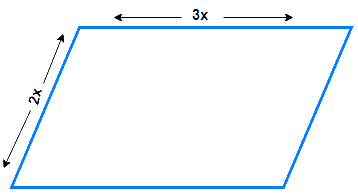# Ex.3.3 Q5 Understanding Quadrilaterals Solution-Ncert Maths Class 8

Go back to  'Ex.3.3'

## Question

The measures of two adjacent angles of a parallelogram are in the ratio $${\rm{3}}:{\rm{2}}$$. Find the measure of each of the angles of the parallelogram.

Video Solution
Ex 3.3 | Question 5

## Text Solution

What is Known?Given figure is a parallelogram and two adjacent angles are having ratio of $${\rm{3}}:{\rm{2}}$$ quadrilateral.

What is Unknown?

Measure of Each angles of parallelogram.

Reasoning:

A parallelogram is a quadrilateral whose opposite angles are equal.

Steps:

We know that the sum of the measures of adjacent angles is $$180º$$ for a parallelogram.

\begin{align}\angle A + \angle B &= {180^{\rm{o}}}\\3x + 2x &= {180^{\rm{o}}}\\5x &= {180^{\rm{o}}}\\x &= \frac{{{{180}^{\rm{o}}}}}{5}\\x &= \,{36^{\rm{o}}}\end{align}

\begin{align} \angle A&=\angle C=3x \\ & ={{108}^{\circ}}\text{ }(\text{ Opposite angles }) \\ \angle B&=\angle D=2x \\ & ={{72}^{\circ}}\text{ (Opposite angles) } \end{align}

Thus, the measures of the angles of the parallelogram are $$108^\circ, 72^\circ, 108^\circ,$$ and $$72^\circ$$.

Learn from the best math teachers and top your exams

• Live one on one classroom and doubt clearing
• Practice worksheets in and after class for conceptual clarity
• Personalized curriculum to keep up with school